# Create a Simple Application using .NET

.Net is a framework for Windows platform for creating web services , business logic oriented application, it is the secondary primary platforms currently being used to develop enterprise solution using web services.

.NET is mainly related with the service oriented architecture.

The .NET framework is a proprietary solution runtime and development platform designed for use with Windows operating systems and server products.

The .NET platform an be used to deliver a variety of applications, ranging from desktop and mobile systems to distributed Web solutions and Web services.

.NET is a Microsoft’s product and in this post i am going to tell how to create a simple application for calculating basic arithmetic operations by using Microsoft Visual Studio 2010.

So we have to first install the Microsoft Visual studio . You can get the Microsoft visual studio by simply clicking this link getintopc.com

Install the Microsoft Visual Studio, it will take some time to install so please wait till the installation finished.

After installation follow the below steps to create a simple calculation application.

In this post i have explained 12 steps to create a simple application using the .Net framework.
Create a .NET Project:
step 1: Open the .NET

Open the Microsoft Visual Studio .NET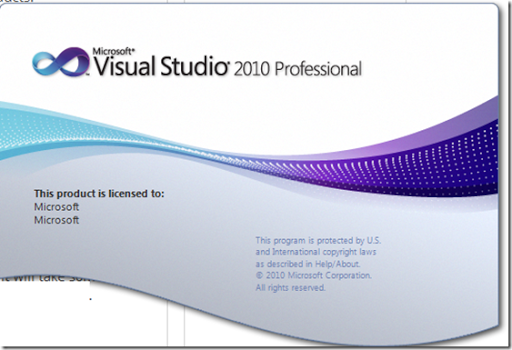step 2:Create a New Project

Open the File and click New—> Project or you can use the short cut key Ctrl+Shift+N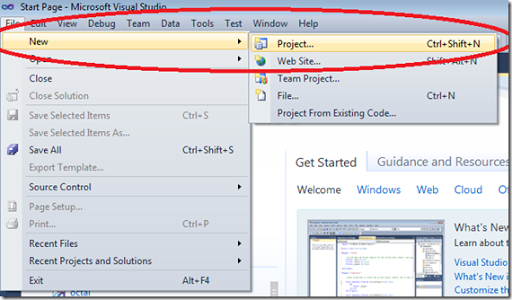step 3:Create a Console Application

Then in the new project window Choose the  Visual C# in the Templates pane and click the Console Application the displayed window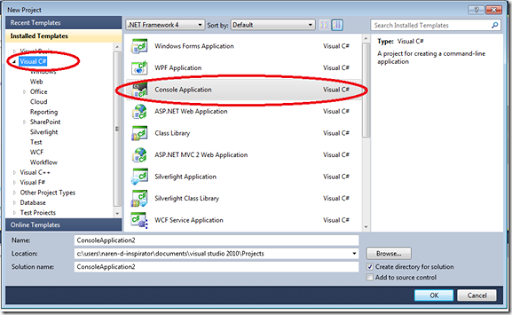then type the project name as Calc and click OK.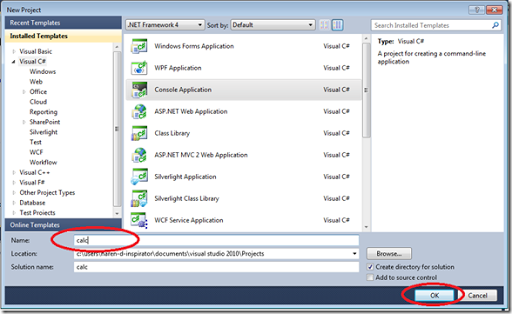Then the project will be loaded for further development of the project .
Then the Coding area will be opened as shown below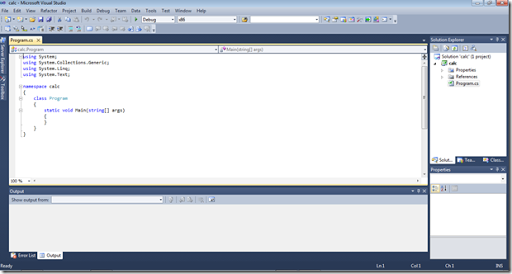Here you can see that there is already some codes on the screen. These are basic codes that are used in every program.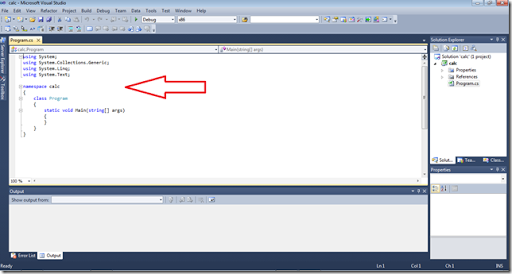In the right side of the window you can see the project calc is opened in the Solution Explorer . If you are unable to see this Solution Explorer you can add this by going to                      view—>Solution Explorer or you can use the short key Ctrl+Shift+L.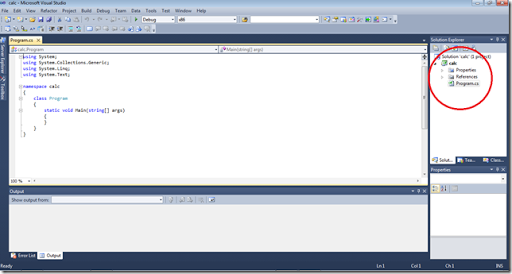In this solution explorer we can able add new classes,and renaming the classes.
step 4: Create new classes
We are now into the coding part. First we need to clarify what are the classes we are going to use , in this example project i am going to use 5 classes including the Main class. The other four classes are add,sub,mul,div we should include the namespaces for these classes in the main class. Let the namespaces for these classes be addition, subtraction,multiplication and division.
Let we create the required classes first then we start coding.
To create a new class go to the solution Explorer and right click the project name Calc and click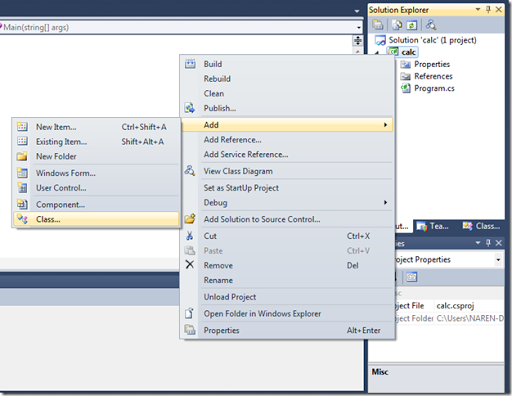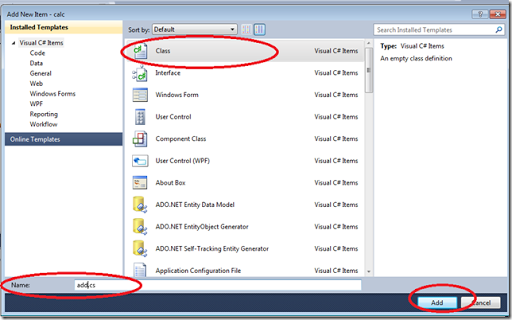Since we are coding in c# the extension of this class is cs.
Thus we have added a new class called add that is used to perform the Addition operation. Now you can see that the class is added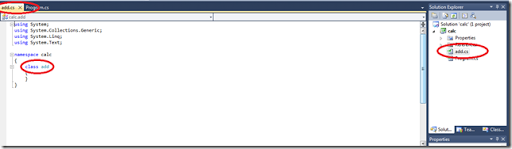We now change the namespace of this class calc to adding.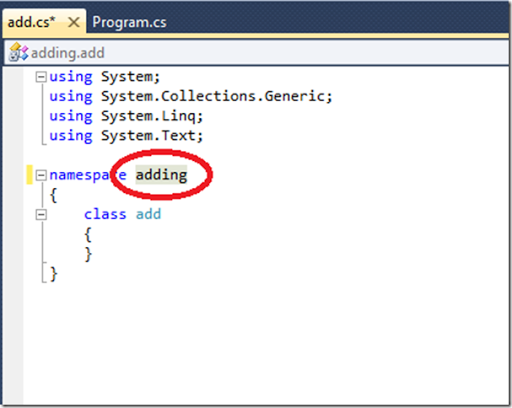Now add this namespace in the program.cs which is the main class as using adding;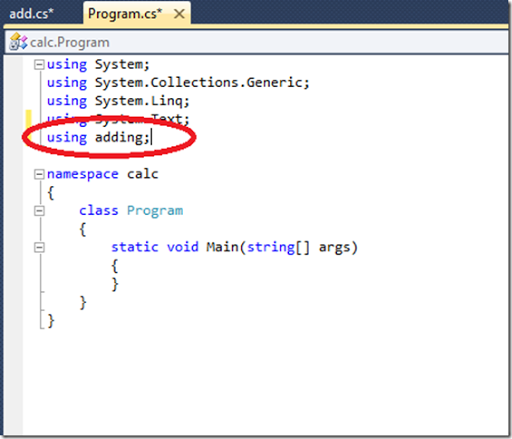Like this we can add the other classes sub, mul ,div and namespaces subtraction , multiplication , division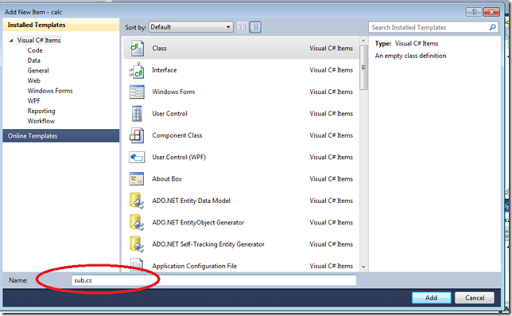Then change the namespace to subtraction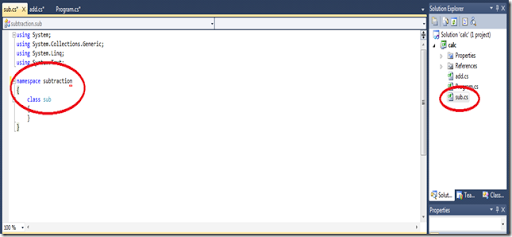Like this add the other two classes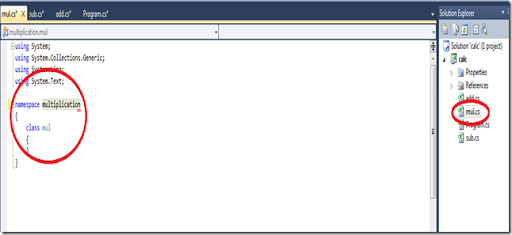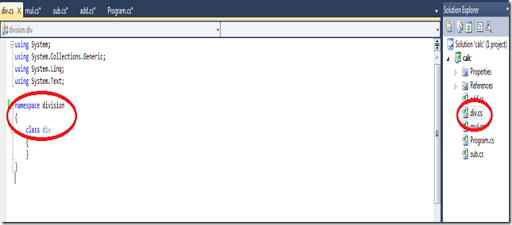Step 5: add namespace in main class
Now ad the namespace in the main class program.cs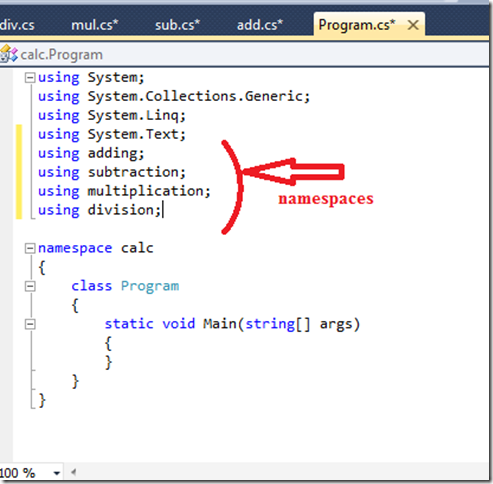That’s it we have now created a skeleton structure for our simple calculator application.

Step 6: Start Coding
Now let we start coding  from the add class…
Just Insert the below code within the class add

{
{
string a,b;
int c,d,e;
Console.WriteLine(“Enter the 1st number:”);
Console.WriteLine(“Enter the 2nd number:”);
c=int.parse(a);
d=int.parse(b);
e=c+d;
Console.WriteLine(”Result={0}”,e);
}
}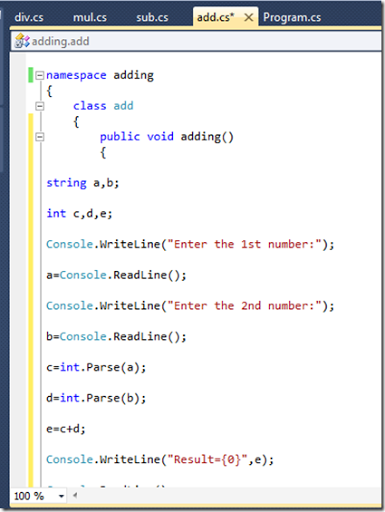Program Explanation:
The c# coding some what similar to the java programming.
Inside the class we have created a method called adding.
in that we have initialized the variable a and b as string type , so that it used to get the input from the user, but the input is integer so we have to convert this string value into the integer by using
integer variable= int.parse(string variable);
In this class we a and b as string type and c,d and e of int type. where c and d are used to store the values from a and b and the e is used to store output value.
If you are familiar with c programming you might have heard the use of printf , it is used to print a statement in the output screen , this work is done in .NET by using the
Console.WriteLine(“statement”);
Then to get the input from the user instead of scanf here we use
in this program we get the two numbers for the calculation a and b  and it is obtained by
to convert we use
c=int.Parse(a);
d=int.Parse(b);
NOTE:while coding , c# is also case sensitive(the combination of lower class and upper class is very important)
to print the output value we use
Console.WriteLine(“Result={0}”,e);
and to make the output screen stable we use
Console.WriteLine();

Step 7: code for other classes also
now code the other classes also, click the sub.cs and type the following code
Program of class sub

namespace subtraction
{
class sub
{
public void subtraction()
{

string a, b;
int c, d, e;
Console.WriteLine("Enter the 1st number:");
Console.WriteLine("Enter the 2nd number:");
c = int.Parse(a);
d = int.Parse(b);
e = c - d;
Console.WriteLine("Result={0}", e);
}
}
}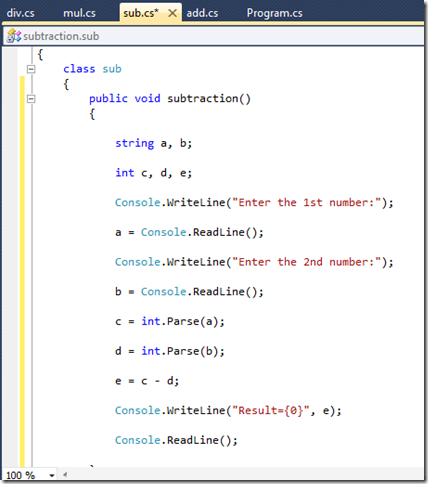Program of class mul:

public void multiplication()
{

string a, b;
int c, d, e;
Console.WriteLine("Enter the 1st number:");
Console.WriteLine("Enter the 2nd number:");
c = int.Parse(a);
d = int.Parse(b);
e = c *d;
Console.WriteLine("Result={0}", e);
}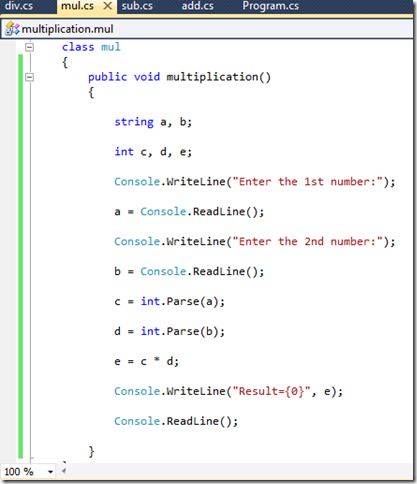Program of class div

public void division()
{

string a, b;
int c, d, e;
Console.WriteLine("Enter the 1st number:");
Console.WriteLine("Enter the 2nd number:");
c = int.Parse(a);
d = int.Parse(b);
e = c / d;
Console.WriteLine("Result={0}", e);
}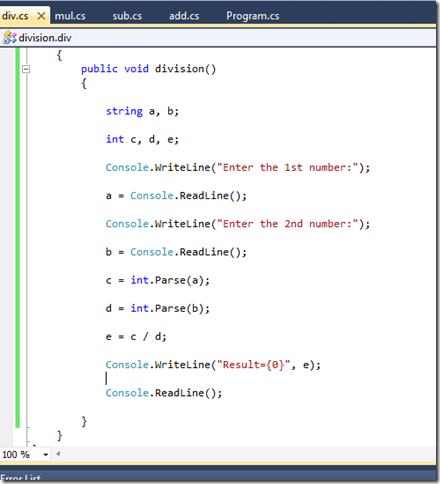step 8: The Main Program
We have finished coding all the other classes now we have to program the main program.
In order to start coding the main program we need to decide how the output screen must be shown to the user .
So , i wish display the list of operations available like this
2.Subtraction
3.Multiplication
4.Division

So, like this the output must be printed, we can print this by using the code Console.WriteLine();
But how we perform to select an option that the user is requested it is by using a concept called as switch case

step 9:switch case statement.
the basic syntax is
switch(option)
{
case 1:
{
…….
…….
…….
}
break;
case 2:
{
…….
…….
…….
}
break;
case n:
{
…….
…….
…….
}
break;
}

By using this we can select the option from the user.
we will first create the baseline of this switch concept for our program
Console.WriteLine(“2.Subtraction”);
Console.WriteLine(“3.Multiplication”);
Console.WriteLine(“4.Division”);
op=int.Parse(h);
switch(op)
{
case 1:
{
}
break;
case 2:
{
Console.WriteLine(“SUBTRACTION”);
}
break;
case 3:
{
Console.WriteLine(“MULTIPLICATION”);
}
break;
case 4:
{
Console.WriteLine(“DIVISION”);
}
break;

Now we have created baseline of the switch case .
Ok now the Concept is simple that,
“ When the user presses the option for example 1 the method ‘adding’ in the class ‘add’ must be invoked to perform the addition operation or if 2 is pressed the method subtraction in the class sub must be invoked to perform the subtraction operation like this other two operations must be performed when the user presses the option   ”

The above concept can be implemented easily by creating an object for each class and using that object we can invoke the methods available.

Step 10: Object creation

In order to understand the concept of object let consider an example Compare the class with a Lock of a house, the methods are the things inside the house and if we need to use those things we need a key to open the lock.
This is the same thing that is happening in the programming.
That key is the object.
By creating an object for a class(house) we can access the methods (things) inside the class.

I think now you are sure about what is an object!!!
That’s good , let we move back to the coding the program !!!
Let me tell how to create an object.

The Syntax for creating an Object is
classname objectname=new classname();
A class is a user defined data type. A data type is the type of the data we are using. For example int a;
In this the ‘int’ is the integer type and ‘a’ is the variable like that class is a data type this type is user defined data type and the object is the variable of the type of class.
The new is keyword that is used to assign the required memory for the class. The new keyword is used to dynamically allocate the memory.

So in case 1
we create the object for the class add
In the above line add is the class name obj1 is the object name , there can be ‘n’ number of objects for a single class.
Like this i create objects for other classes also..

switch(op)
{
case 1:
{
}
break;
case 2:
{
Console.WriteLine(“SUBTRACTION”);
sub obj2=new sub();
}
break;
case 3:
{
Console.WriteLine(“MULTIPLICATION”);
mul obj3=new mul();
}
break;
case 4:
{
Console.WriteLine(“DIVISION”);
div obj4=new div();
}
break;

Step 11: Access the methods
Then our next step to access the methods from the classes
the syntax is
object name.methodname();

switch(op)
{
case 1:
{
}
break;
case 2:
{
Console.WriteLine(“SUBTRACTION”);
sub obj2=new sub();
obj2.subtraction()
}
break;
case 3:
{
Console.WriteLine(“MULTIPLICATION”);
mul obj3=new mul();
obj3.multiplication();
}
break;
case 4:
{
Console.WriteLine(“DIVISION”);
div obj4=new div();
obj4.division();
}
break;

I think we have finished the coding let we check it
Program.cs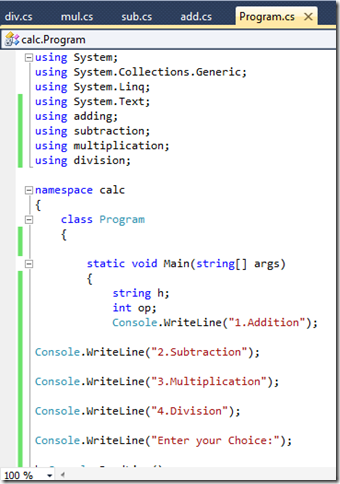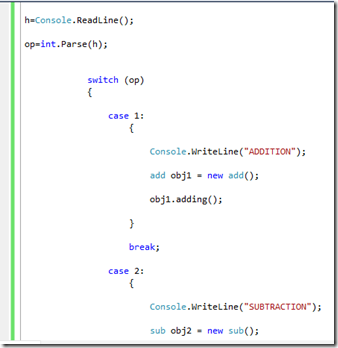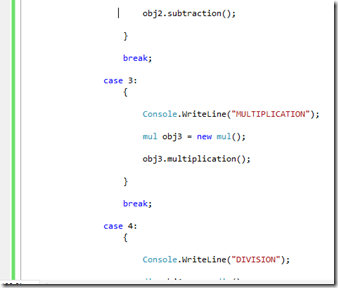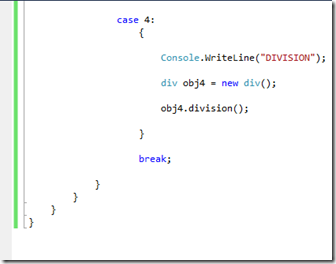I also add another case 5: for exit option

case 5:
{
}
break;
That’s all guys we have done the coding part now we have to just run the application .

Step 12: Run the Application
To run the program just click the RUN button in the TOOLBAR.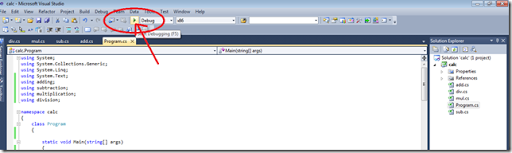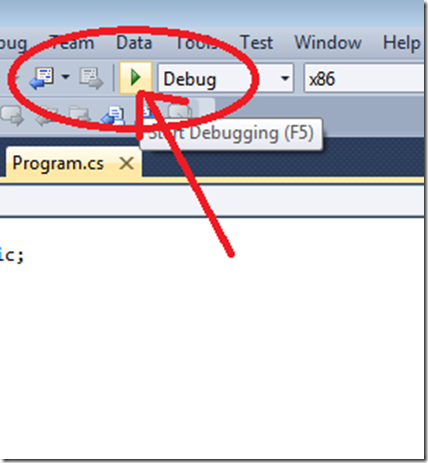Click it and you will get the output
Output Screen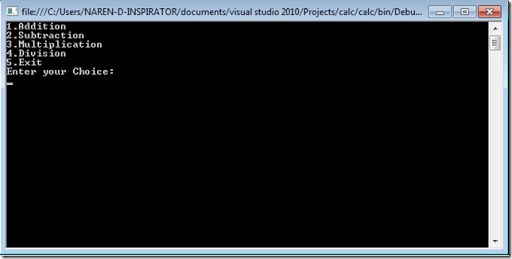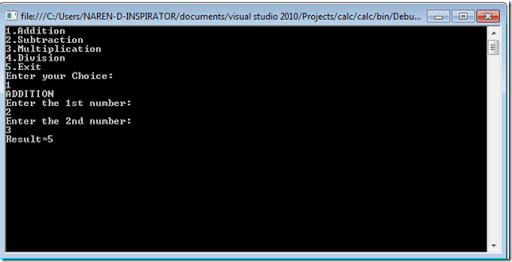Output – Subtraction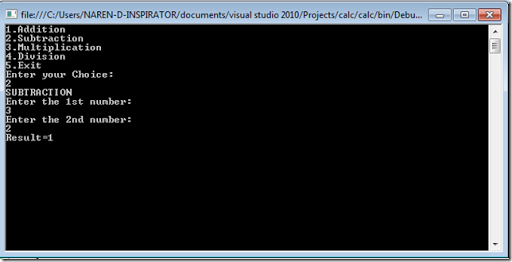Output- Multiplication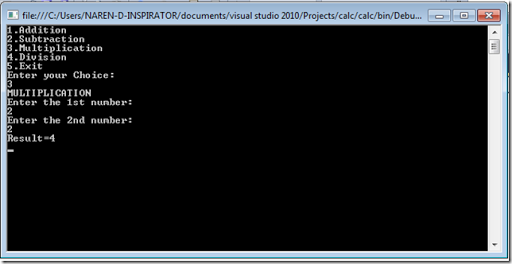Output- Division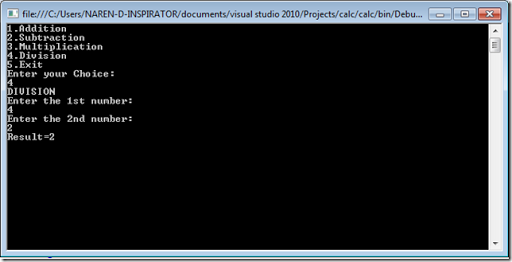Output-Exit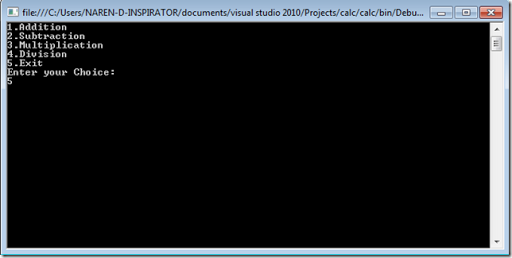Output-Terminated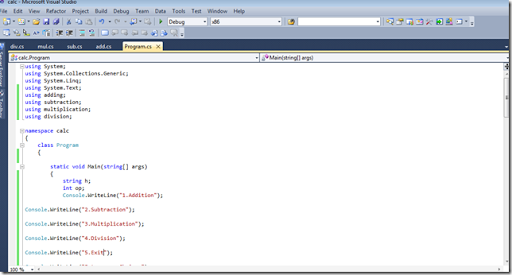Congratulations !!!!
We have successfully created a calculator application using .NET .
Share the post if you Like ….